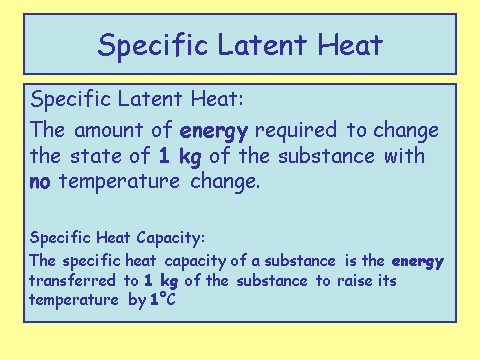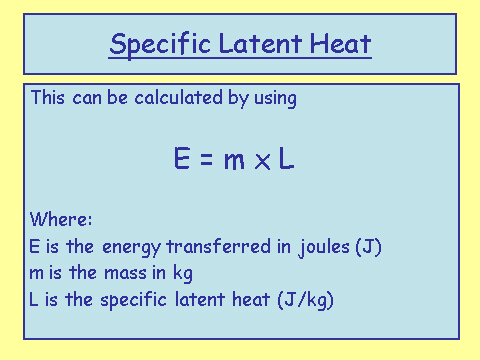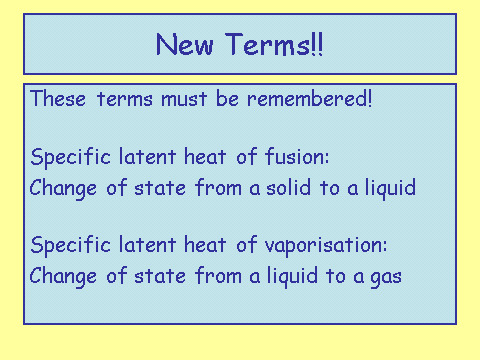# Specific Latent Heat

## Define Specific Latent HeatI also included the definition for specific heat capacity underneath specific latent heat for comparison.

In less words: specific latent heat is the measure of how much energy is required to change the state of a substance. However, in the exam you must use the definition in the slide above.

## Specific Latent Heat EquationSometimes E is also written as Q.

This equation is provided to you in an exam, but you still need to learn it and know how to apply it.

There are two ‘types’ of latent heat. These occur when a substance is melting/freezing and condensing/boiling. The specific terms are shown below. It is important that you learn them so you know which constants to apply in a given question.The specific latent heat of fusion is always a smaller value than vaporisation. This is because the particles travel a smaller distance when changing from a solid to a liquid, than when they change from a liquid to a gas.

## Using the Specific Latent Heat EquationWorked examples:

Calculate the energy released when 100 g of steam condenses.

__Step 1: __Identify what you know and convert to standard units

``````Mass  100 g =  0.1 kg

Since it is a change of state between gas and liquid you will need to use the latent heat of vaporisation.

Latent heat of vaporisation = 2 260 000 J/Kg
``````

Step 2: Substitute the numbers into the equation

``````Energy required = mass x specific latent heat of vaporisation

0.1 x  2 260 000

E = 226 000 J
``````

Calculate the mass of ice lost from an iceberg melting, if the energy supplied was 835 000 000J.

Step 1: Identify what you know and convert to standard units

``````Energy = 835 000 000J

Since it is a change of state between a solid and a liquid, you will need to use the latent heat of fusion.

Latent heat of fusion = 334 000 J/Kg
``````

Step 2: Substitute the numbers into the equation

``````Energy required = mass x specific latent heat

835 000 000 J = mass x 334 000
``````

Step 3 Rearrange the equation to make mass the subject of the equation.

``````Divide both sides of the equation by 334 000

Mass = 2500 kg
``````

Specific latent heat and specific heat capacity go together really well for five mark questions since they both have energy, mass and are related to each other as they are in the same topic.

Here is an example; try to do it yourself before following the working. Use values from the table above.

Q. Calculate the mass of water you could evaporate from its boiling point with the energy required to melt 800 g of silver from its melting point.

A. Energy required to melt silver:

``````Energy = mass x latent heat of fusion

Energy = 0.8 x 88 000	(1)

Energy = 70 400 J	(1)

Mass of water that can be evaporated:

Energy = mass x Latent heat of vaporisation

70 400 = mass x 2 260 000	(1)

Mass = 70 400/ 2 260 000 	(1)

Mass = 0.031 kg	(1)
``````